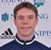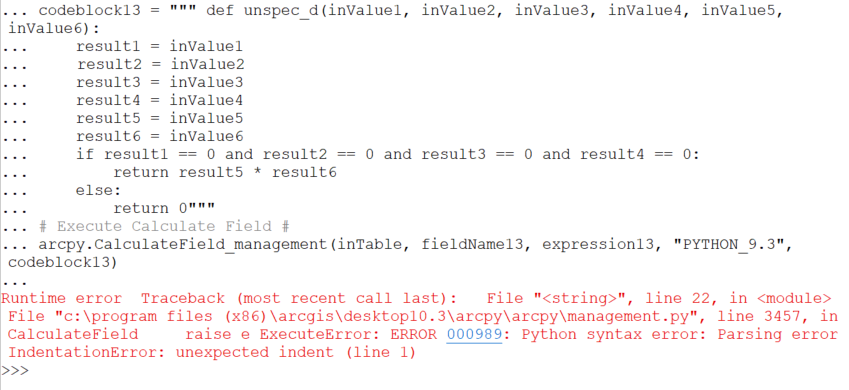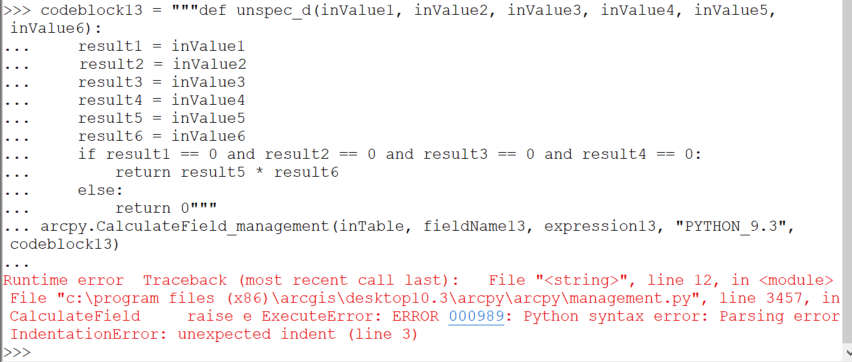# Help with syntax error in Calculate Field tool

2182
10
07-29-2020 07:34 AMNew Contributor II

Hi Esri community, I am getting an error for a script I've written to calculate several fields. The error message says there is a syntax error, but I have tried to fix it and I don't know what I've done wrong. The error message is as follows:

Runtime error Traceback (most recent call last): File "<string>", line 97, in <module> File "c:\program files (x86)\arcgis\desktop10.3\arcpy\arcpy\management.py", line 3457, in CalculateField raise e ExecuteError: ERROR 000989: Python syntax error: Parsing error IndentationError: unexpected indent (line 1)

The script I used worked for every field except for the last one, which was the only field that needed codeblock to calculate. The portion of the script for the last field is as follows (line 97 is the last line) :

# Import system modules #
import arcpy
# Set environment settings #
arcpy.env.workspace = r"D:\mapsfolder"

# Set local variables for unassigned #
inTable = r"D:\mapsfolder\table.shp"
fieldName13 = "unassigned"
expression13 = "unassigned_fxn(!type1year!,!type2year!,!type1end!,!type2end!,!eventyear!,!occurrence!)"
codeblock13 = " " " def unassigned_fxn(inValue1, inValue2, inValue3, inValue4, inValue5, inValue6):
result1 = inValue1
result2 = inValue2
result3 = inValue3
result4 = inValue4
result5 = inValue5
result6 = inValue6
if result1 == 0 and result2 == 0 and result3 == 0 and result4 == 0:
return result5 * result6
else:
return 0" " "
# Execute Calculate Field #
arcpy.CalculateField_management(inTable, fieldName13, expression13, "PYTHON_9.3", codeblock13)

Thanks for any help!!

1 Solution

Accepted SolutionsbyMVP Esteemed Contributor

codeblock = 'def unassigned_fxn(inValue1, inValue2, inValue3, inValue4, inValue5, inValue6):\n    result1 = inValue1\n    result2 = inValue2\n    result3 = inValue3\n    result4 = inValue4\n    result5 = inValue5\n    result6 = inValue6\n    if result1 == 0 and result2 == 0 and result3 == 0 and result4 == 0:\n        return result5 * result6\n    else:\n        return 0'

there was an extra space in your return sections

... sort of retired...
10 RepliesbyMVP Esteemed Contributor

You need to indent everything within a function definition.  What you have now is the same as:

``````def unassigned_fxn(inValue1, inValue2, inValue3, inValue4, inValue5, inValue6):
result1 = inValue1
result2 = inValue2
....‍‍‍‍``````

and what you need is:

``````def unassigned_fxn(inValue1, inValue2, inValue3, inValue4, inValue5, inValue6):
result1 = inValue1
result2 = inValue2
....‍‍‍‍``````byMVP Esteemed Contributor

Notice how \n is inserted where appropriate and the indentation is maintained.

A 'print' statement will confirm everything

``````codeblock13 = """def unassigned_fxn(inValue1, inValue2, inValue3, inValue4, inValue5, inValue6):
result1 = inValue1
result2 = inValue2
result3 = inValue3
result4 = inValue4
result5 = inValue5
result6 = inValue6
if result1 == 0 and result2 == 0 and result3 == 0 and result4 == 0:
return result5 * result6
else:
return 0"""

codeblock13

'def unassigned_fxn(inValue1, inValue2, inValue3, inValue4, inValue5, inValue6):\n    result1 = inValue1\n    result2 = inValue2\n    result3 = inValue3\n    result4 = inValue4\n    result5 = inValue5\n    result6 = inValue6\n    if result1 == 0 and result2 == 0 and result3 == 0 and result4 == 0:\n         return result5 * result6\n    else:\n         return 0'

print(codeblock13)

def unassigned_fxn(inValue1, inValue2, inValue3, inValue4, inValue5, inValue6):
result1 = inValue1
result2 = inValue2
result3 = inValue3
result4 = inValue4
result5 = inValue5
result6 = inValue6
if result1 == 0 and result2 == 0 and result3 == 0 and result4 == 0:
return result5 * result6
else:
return 0‍‍‍‍‍‍‍‍‍‍‍‍‍‍‍‍‍‍‍‍‍‍‍‍‍‍‍‍‍``````

... sort of retired...New Contributor II

Thanks for both of your help!

I am still getting an error saying I need an indent even when fix what you mentioned.... not sure why, especially when the code works when I use it in field calculator.byMVP Esteemed Contributor

you have a space after the triple quote... remove it

... sort of retired...New Contributor II

Thanks for the help! unfortunately I am still getting an error message:byMVP Esteemed Contributor

Look closely at 'result2 = inValue2' ; to me it looks like it's in one space extra. Compare how the r's line up above that line and below... (Using the syntax highliter rather than a screen shot would help...)

That should just about do it....byMVP Esteemed Contributor

codeblock = 'def unassigned_fxn(inValue1, inValue2, inValue3, inValue4, inValue5, inValue6):\n    result1 = inValue1\n    result2 = inValue2\n    result3 = inValue3\n    result4 = inValue4\n    result5 = inValue5\n    result6 = inValue6\n    if result1 == 0 and result2 == 0 and result3 == 0 and result4 == 0:\n        return result5 * result6\n    else:\n        return 0'

there was an extra space in your return sections

... sort of retired...New Contributor II

Thanks that fixed the problem and it finally worked!!

Thanks to everybody who helped!New Contributor II

Alternatively, if you don't want the headache of getting the syntax perfect inside a big triple quote string, you can use an update cursor.

Something like this:

``````inTable = r"D:\mapsfolder\table.shp"
field_list = ["type1year","type2year","type1end","type2end","eventyear","occurrence", "unassigned"]
with arcpy.da.UpdateCursor(inTable, field_list) as cursor:
for row in cursor:
# Using any(), if any of those fields are not zero, this will execute.
if any([row, row, row, row]):
result = row * row
else:
result = 0

row = result
cursor.updateRow(row)‍‍‍‍‍‍‍‍‍‍‍‍``````

You also get more control of the values each field is returning and can deal with them more deftly.